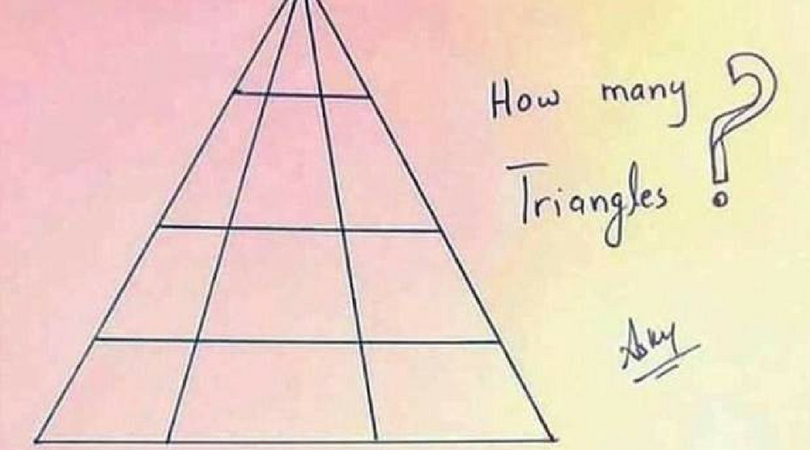Kumar Ankit

Calling all optical puzzlers/ math experts/ brainteaser aficionados! Are you ready to make your brain hurt? That’s exactly what this triangle puzzle will do, and let me tell you, it isn’t fun. The seemingly ‘simple’ new brainteaser asks one to calculate the number of triangles in a picture, leaving the Internet, and me, baffled by the answer.

The drawing is simple. Created by Doctor ASKY, also known as Kumar Ankit, it has been shared over a hundred thousand times on Facebook. Ankit is an engineering student from India, and designed this geometric puzzle, posting it to Quora to see if people understand the logical explanation and reason behind it. What is so bizarre about this is that it is just a simple sketch, a dumb sketch on a paper, that asks users to calculate how many physical triangles it contains. Take a look at it below. Can you figure out the correct answer?

The Internet has been left very dumbfounded by the simple question, with answers ranging from 4 triangles to 44 triangles. Yes, 44 triangles. Users have taken to the comments section to share their calculation, and let’s just say, I don’t’ feel so bad about myself.

But, in case you were wondering, the total number is 25. There are 24 triangles contained within the main triangle, as well as one little sneaky triangle in the artist’s signature, making it the 25th triangle.

If you’re about to throw your computer because you don’t understand how this is possible, it’s okay, it’s simple to work it out once you actually know the strategy behind it. Like everything in life, there is an answer to all questions. Except for the Bermuda Triangle.

You see, there are six triangles in the top row- three individuals, two pairs, and one triangle made up of all three. Then there are six in the second row, six in the third row, and six in the fourth row using the same exact strategy! Then of course, the small triangle in the letter A in the signature. Simple, huh? No? Okay, maybe this will help.

This little diagram that actually explained it all, was created by mathematician Martin Silvertant. He created to illustrate how he reached 25, which let’s face it, is much much easier. Who knew it would be that simple.About the author: